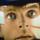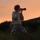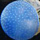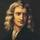## General Question# About the proton impact velocity at LHC?

Asked by gciochina (107) August 11th, 2008

This one might sound silly: I’ve read about the Large Hadron Collider and i was wondering: There we have two beams of protons (travelling at 99.99% of the speed of light) that collide head on. So talking about a perfect collision (max velocity and head on collision) isn’t the impact speed greater that the speed of light? Isn’t this impossible in our universe? Sorry again if the question sounds stupid…

Observing members: 0Composing members: 0No its not, When the two particles collide it creates an explosion of epic proportions. The purpose of a proton collider is to recreate events of the ‘Big Bang’ when new matter was made out of smaller amounts, seemingly impossible. By recreating these events scientists can learn more about the origin of our universe. So to answer your question the impact speed is not greater because they collide head on canceling out each others velocity rather than combing it to a speed greater than that of light.

joeysefika (3093)“Great Answer” (0) Flag as…This question got me thinking! I mean, the speed relative to each other must me a lot greater than the speed of light!

Magnus (2884)“Great Answer” (0) Flag as…This is an excellent question. To understand the answer, you need to know a bit about Einstein’s Special Theory of Relativity. In classical physics, if two particles are traveling in opposite directions at 90% of the speed of light, their relative velocity is obtained by simple addition: 90% + 90% = 180% or 0.9 + 0.9 = 1.8 times the speed of light. In relativity, many strange things happen, one of which is that you cannot add velocities this way. The correct relative velocity is: (0.9+0.9)/(1+0.9*0.9)=0.9945 or 99.45% of the speed of light. When the velocity of each particle is 99.99%, the relative velocity is 99.9999995%.

So, the relative velocity always gets closer to the speed of light, but never exceeds it. The mathematics of this is actually rather simple, but understanding the weird changes in our classical conception of space and time is the part that is both difficult and extremely fascinating.

JWTK (49)“Great Answer” (4) Flag as…or